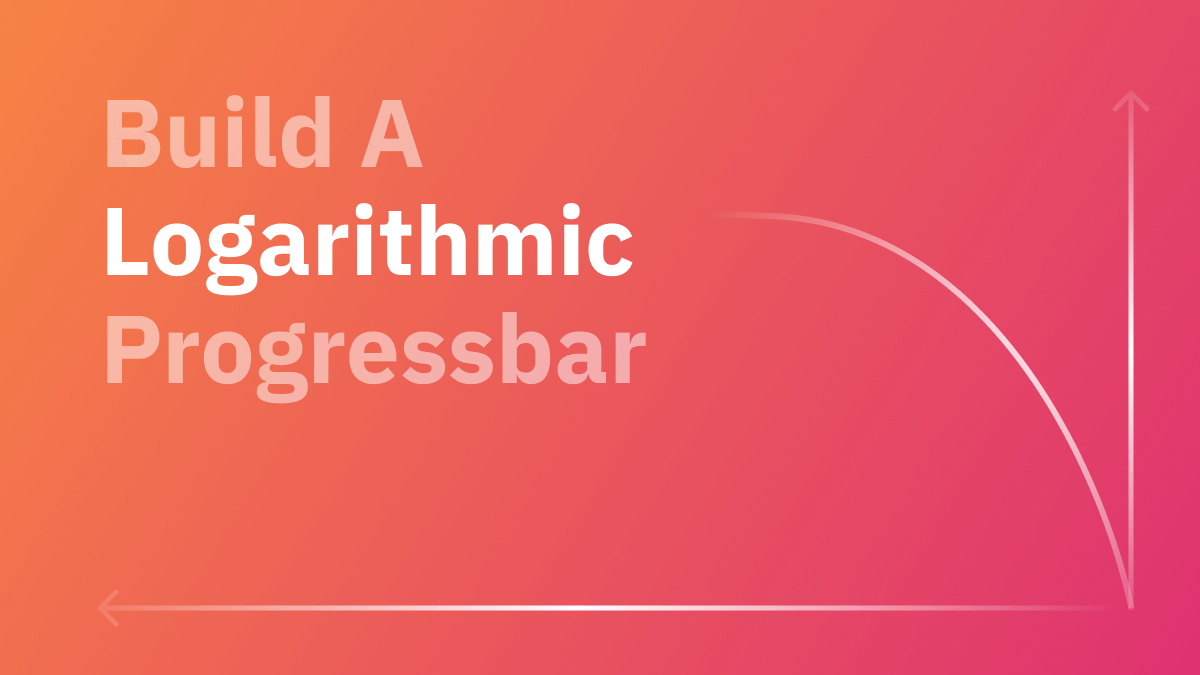# Build a Logarithmic Progress Bar

November 21st, 2022 . 4 min read

When we build progress bar to track progress of any event or task, we often build a linear progress bar. A linear progress bar means that the bar increases proportionally with the progress of the event i.e for 25% progress the bar will show 25% completed.( You can see a linear progress bar at the top of this very website as you keep scrolling down the article😉 ) Pretty straightforward right? You might be thinking "Psst! That's how a progress bar works" and you are right but... should you always use it? Is there a better way to build a progress bar?

Just want to see the code? Jump here.

## Logarithmic Progress Bar: What and Why?

Let's say you built an app which asks the user to perform multiple tasks, maybe its a series of important onboarding steps or a survey. With each step performed by the user, the progress bar... well progresses! Progress bars are great for users to see how far they've come and at times they encourage users to complete the whole series of tasks. But if you have a lot of steps and the user sees that they haven't made much progress in the beginning few steps, they might feel unmovitivated to continue further. What if we could encourage users by showing them more progress for the starting steps. This is where logarithmic progress bar comes in.

In the example below try adjusting the progress slider from the beginning to the end and see how the linear and logarithmic progressbar behave.

Linear:
Logarithmic:

As you can see, the linear progress bar changes "linearly" with the slider but the logarithmic progressbar progresses much more in the beginning. This might be what we need in many cases to motivate the users early on.

## The Math

I know! I know! Maths is boring right? I hate maths most of the times too but bear with me. When you build a linear progress bar, the basic math is:

const progress = (current_step / total_steps) * 100;


For a logarithmic progress bar we will change this a bit. We will calculate the progress using the logarithmic function i.e.

$log{_b}{a}$

Our current_step will be the argument(a) and total_steps will be the base(b). So calculating the value of this logarithmic function will give us the progress. We do have a Math.log function in JavaScript but that only calculates the natural logarithm i.e. log with base e( Euler's number ). Luckily we have a formula to calculate our required function.

$\log{_b}{a} = \log{_e}{a} / \log{_e}{b}$

So our code will look like:

const getBaseLog = (base, arg) => {
return Math.log(arg) / Math.log(base);
};

const progress = Math.round(getBaseLog(total_steps, current_step)) * 100;


## Building the component

Now that we have figured out the math, it's much more easier to create the component. I will be using React and Tailwind.

progress-bar.jsx
const ProgressBar = ({ current_val, max_val }) => {
const getBaseLog = (base, arg) => {
return Math.log(arg) / Math.log(base);
};

return (
<div
style={{
width: Math.round(getBaseLog(max_val, current_val) * 100) + '%',
}}
className="h-5 bg-lime-500"
></div>
);
};


## Conclusion

When I first discovered about logarithmic progressbars, I was both impressed and concerned at the same time. I thought "Isn't this a bit misleading to the user?" A bit! Yes, but from my experience in both design and development, I have come to realize that sometimes perfection, accuracy and ethics need to be thrown out of the window for better user experience because human minds don't perceive things as they are. You might have seen visual illusions where exactly same colored or same sized objects appear different. I like to see the logarithmic progressbar as an improved user experience compared to linear progressbars.

Back to the blog